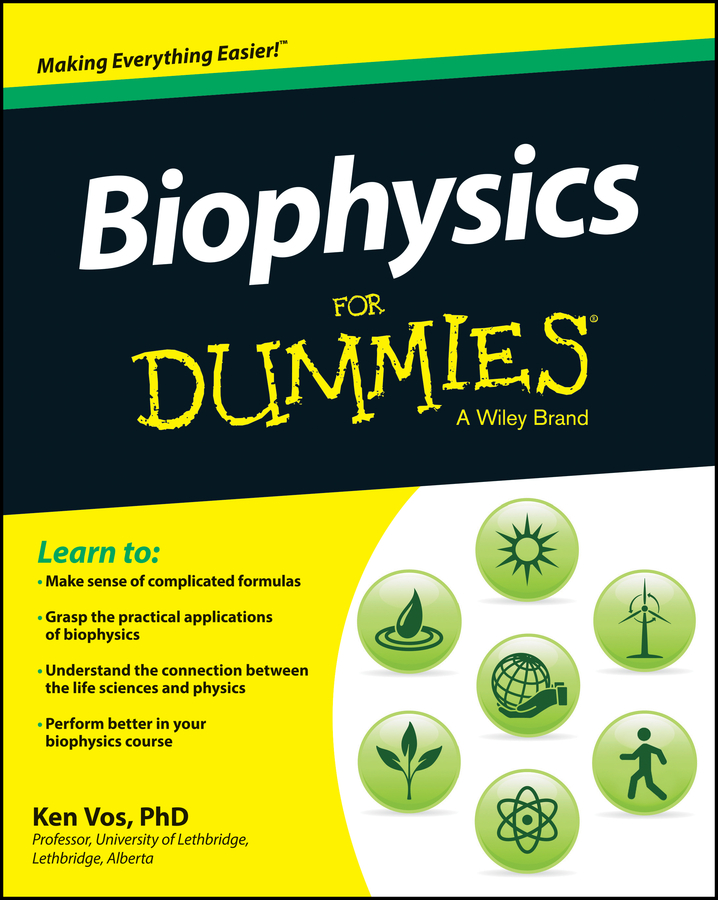##### Biophysics For DummiesBiomechanics, which is the study of the interaction of biological organisms with their surroundings, is an important area of biophysics. A solid understanding of biomechanics is important for people interested in kinesiology, physiotherapy, occupational therapy, and bioengineering. Biomechanics also deals with the small objects, such as the interaction of cells with their surroundings.

Solving problems in biomechanics involves three important steps:

1. Draw a figure, graph, or free-body diagram, even if a picture is provided.

Visualizing what you know is a very important first step.

2. Make lists and tables of what you know.

Having a clear picture of what you know makes it easier to plot a path to the solution.

3. Apply the appropriate physical principle.

Some of the important concepts in biomechanics are

• The three fundamental types of energy used in biomechanics are kinetic energy, potential energy, and heat energy. The other two fundamental types of energy (not used in biomechanics) are mass energy and electromagnetic radiation.

• The connection between forces, torques, work, and power.

• The work-energy theorem equating the change in the kinetic energy to the net work done.

• The conservation of total energy, which means you can’t create or destroy energy.

• The conservation of (linear/angular) momentum in an isolated system. Conservation of linear momentum means the velocity of the center of mass is either zero or constant.

• Newton’s three laws of motion, which tell you how forces influence objects and change their motion.

• Kinematics gives the relationship between position, velocity, and acceleration, which allows you to describe the motion of an object without worrying about what is causing the motion.